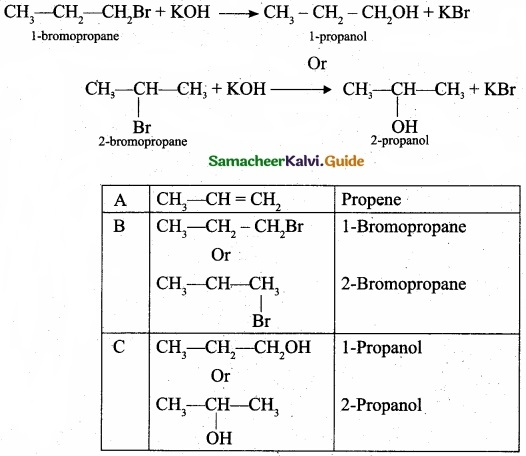Students can Download Tamil Nadu 11th Chemistry Model Question Paper 2 English Medium Pdf, Tamil Nadu 11th Chemistry Model Question Papers helps you to revise the complete Tamilnadu State Board New Syllabus, helps students complete homework assignments and to score high marks in board exams.

## TN State Board 11th Chemistry Model Question Paper 2 English Medium

General Instructions:

1. The question paper comprises of four parts.
2. You are to attempt all the parts. An internal choice of questions is provided wherever applicable.
3. All questions of Part I, II, III and IV are to be attempted separately.
4. Question numbers 1 to 15 in Part I are Multiple Choice Questions of one mark each.
These are to be answered by choosing the most suitable answer from the given four alternatives and writing the option code and the corresponding answer
5. Question numbers 16 to 24 in Part II are two-mark questions. These are to be answered in about one or two sentences.
6. Question numbers 25 to 33 in Part III are three-mark questions. These are to be answered in about three to five short sentences.
7. Question numbers 34 to 38 in Part IV are five-mark questions. These are to be answered in detail Draw diagrams wherever necessary.

Time: 2:30 Hours
Maximum Marks: 70

PART – I

Answer all the Questions: [15 × 1 = 15]
Choose the most suitable answer from the given four alternatives.

Question 1.
An element X has the following isotopic composition 200X = 90 %, 100X = 8 % and 202X = 2 %.
The weighted average atomic mass of the element X is closest to ………………………….
(a) 201 u
(b) 202 u
(c) 199 u
(d) 200 u
$$\frac { (200\times 90)+(199\times 18)+(202\times 2) }{ 100 }$$
= 199.96 = 200 u
(d) 200 uQuestion 2.
Which of the following is not used in writing electronic configuration of an atom?
(a) Aufbau principle
(b) Hund’s rule
(c) Pauli’s exclusion principle
(d) Heisenberg’s uncertainty principle
(d) Heisenberg’s uncertainty principle

Question 3.
Assertion (A): Cr with electronic configuration [Ar]3d5 4s1 is more stable than [Ar] 3d4 4s2.
Reason (R): Half filled orbitals have been found to have extra stability than partially filled orbitals.
(a) A and R are correct and R is the correct explanation of A.
(b) A and R are correct but R is not the correct explanation of A.
(c) A is correct but R is wrong.
(d) A is wrong but R is correct.
(a) A and R are correct and R is the correct explanation of A.Question 4.
The type of H-bonding present in ortho nitro phenol and p-nitro phenol are respectively ……………………..
(a) Inter molecular H-bonding and intra molecular H – bonding
(b) Intra molecular H-bonding and inter molecular H – bonding
(c) Intra molecular H – bonding and no H – bonding
(d) Intra molecular H – bonding and intra molecular H – bonding(b) Intra molecular H-bonding and inter molecular H – bonding

Question 5.
Match the following: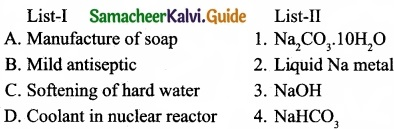Question 6.
Which of the following is the correct expression for the equation of state of van der Waals gas?
(a) $$\left(P+\frac{a}{n^{2} V^{2}}\right)$$ (V – nb) = nRT
(b) $$\left(P+\frac{n a}{n^{2} V^{2}}\right)$$ (V – nb) = nRT
(c) $$\left(P+\frac{a n^{2}}{V^{2}}\right)$$ (V – nb) = nRT
(d) $$\left(P+\frac{n^{2} a^{2}}{V^{2}}\right)$$ (V – nb) = nRT
(c) $$\left(P+\frac{a n^{2}}{V^{2}}\right)$$ (V – nb) = nRTQuestion 7.
In a reversible process, the change in entropy of the universe is ………………………
(a) > 0
(b) >0
(c) <0
(d) = 0
(d) = 0

Question 8.
Which of the following is not a general characteristic of equilibrium involving physical process?
(a) Equilibrium is possible only in a closed system at a given temperature
(b) The opposing processes occur at the same rate and there is a dynamic but stable condition
(c) All the physical processes stop at equilibrium
(d) All measurable properties of the system remains constant
Solution:
Correct statement: Physical processes occurs at the same rate at equilibrium.
(c) All the physical processes stop at equilibrium

Question 9.
Stomach acid, a dilute solution of HCl can be neutralised by reaction with Aluminium hydroxide
Al(OH)3 + 3HCl(aq) → AlCl3 + 3H2O
How many millilitres of 0.1 M Al(OH)3 solution are needed to neutralise 21 mL of 0.1 M HCl?
(a) 14 mL
(b) 7 mL
(c) 21 mL
(d) None of these
Solution:
M1 × V = M2 × V2
∵ 0.1 M Al(OH)3 gives 3 × 0.1 = 0.3 M OH ions
0.3 × V1 = 0.1 × 21
V1 = $$\frac{0.1×21}{0.3}$$ = 7 ml
(b) 7 mLQuestion 10.
Shape and hybridisation of IF5 are ……………………..
(a) Trigonal bipyramidal, sp3d2
(b) Trigonal bipyramidal, sp3d
(c) Square pyramidal, sp3d2
(d) Octahedral, sp3d2
Solution:
IF5 – 5 bond pair + 1 lone pair
∴ hybridisation sp3d2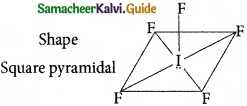(c) Square pyramidal, sp3d2

Question 11.
Consider the following statements:

1. It is not possible for the carbon to form either C4+ (or) C4- ions.
2. Carbon can form ionic bonds.
3. In compounds of carbon, it form covalent bonds.

Which of the above statement is/are not correct?
(a) (I) and (II)
(b) (III) only
(c) (I) only
(d) (II) only
(d) (II) onlyQuestion 12.
For the following reactions
(A) CH3CH2CH2Br + KOH → CH3-CH + KBr + H2O
(B) (CH3)3CBr + KOH → (CH3)3 COH + KBr
(C)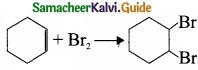Which of the following statement is correct?
(a) (A) is elimination, (B) and (G) are substitution
(b) (A) is substitution, (B) and (C) are elimination
(c) (A) and (B) are elimination and (C) is addition reaction
(d) (A) is elimination, B is substitution and (C) is addition reaction.
(d) (A) is elimination, B is substitution and (C) is addition reaction.

Question 13.
Which of the following compound used for metal cleaning solvent?
(a) Methylene chloride
(b) Methyl chloride
(c) Chloroform
(d) Ethane
(a) Methylene chlorideQuestion 14.
The number of possible isomers of C6H12 is ……………………..
(a) 2
(b) 3
(c) 5
(d) 6
(c) 5

Question 15.
Ozone layer is depleted by the reactive ……………………..
(a) Hydrogen atom
(b) Oxygen atom
(c) Fluorine atom
(d) Chlorine atom
(d) Chlorine atom

PART – II

Answer any six questions in which question No. 19 is compulsory. [6 × 2 = 12]

Question 16.
Calculate the average atomic mass of naturally occurring magnesium using the following data?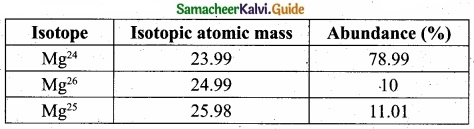Solution: Isotopes of Mg.
Atomic mass = Mg24 = 23.99 × 78.99/100 = 18.95
Atomic mass = Mg26 = 24.99 × 10/100 = 2.499
Atomic mass = Mg25 = 25.98 × 11.01/100 = 2,860
Average Atomic mass = 24.309
Average atomic mass of Mg = 24.309Question 17.
What are quantum numbers?

1. The electron in an atom can be characterized by a set of four quantum numbers, namely – principal quantum number (n), azimuthal quantum number (l), magnetic quantum number (m) and spin quantum number (s).
2. When Schrodinger equation is solved for a wave function φ, the solution contains the first three quantum numbers n, 1 and m.
3. The fourth quantum number arises due to the spinning of the electron about its own axis.

Question 18.
How 2-ethylanthraquinone helps to prepare hydrogen peroxide?
On an industrial scale, hydrogen peroxide is prepared by auto oxidation of 2-alkyl anthraquinol.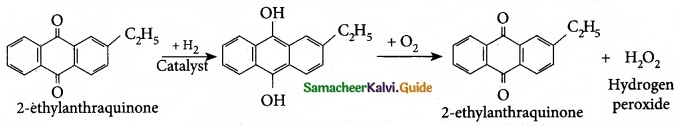Question 19.
Calculate the pressure exerted by 2 moles of sulphur hexafluoride in a steel vessel of volume 6 dm3 at 70°C assuming it is an ideal gas?
We will use the ideal gas equation for this calculation as below:
P = $$\frac{nRT}{V}$$ = image 7 = 9.39 atm.Question 20.
What are the important features of lattice enthalpy?

1. Higher lattice energy shows greater electrostatic attraction and therefore a stronger bond in the solid.
2. The lattice enthalpy is greater for ions of higher charge and smaller radii.

Question 21.
What are aqueous and non-aqueous solution? Give example?

1. If the solute is dissolved in the solvent water, the resultant solution is called as an aqueous solution, e.g., salt in water.
2. If the solute is dissolved in the solvent other than water such as benzene, ether, CCl4 etc, the resultant solution is called a non aqueous solution, e.g., Br2 in CCl4.

Question 22.
What is bond enthalpy? How they relate with bond strength?
The bond enthalpy is defined as the minimum amount of energy required to break one mole of a particular bond in molecules in their gaseous state. Larger the bond enthalpy stronger will be the bond.Question 23.
What is triad system? Give example?
(I) In this system hydrogen atom oscillates between three polyvalent atoms. It involves 1, 3 – migration of hydrogen atom from one polyvalent atom to other with in the molecule

(II) The most important type of triad system is keto-enol tautomerism and the two groups of tautomers are keto form and enol form.

(III)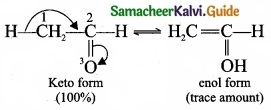Question 24.
What is stone leprosy? How is it formed?

1. The attack on, the marble of buildings by acid rain is called stone leprosy.
2. Acid rain causes extensive damage to buildings made up of marble.

CaCO + H2SO4 → CaSO4 + H2O + CO2

PART – III

Answer any six questions in which question No. 26 is compulsory. [6 × 3 = 18]

Question 25.
Calculate the equivalent mass of hydrated ferrous sulphate?
Hydrated ferrous sulphate = FeSO4.7H2O
Ferrous sulphate – Reducing agent
Ferrous sulphate reacts with an oxidising agent in acid medium according to the equation.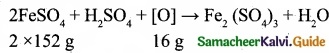16 parts by mass of oxygen oxidised 304 g of FeSO4.
8 parts by mass of oxygen will oxidise $$\frac{304}{16}$$ × 8 parts by mass of FeSO4 = 152
Equivalent mass of Ferrous sulphate (Anhydrous) = 152
Equivalent mass of crystalline Ferrous sulphate FeSO4.7H2O = 152 + 126 = 278Question 26.
Calculate the uncertainty in position of an electron, if ∆v = 0.1% and v = 2.2 × 106 ms-1.
Mass of an electron = m = 9.1 × 10-31 kg.
∆v = Uncertainty in velocity = $$\frac{0.1}{100}$$ × 2.2 × 106 ms-1
∆v = 0.22 × 104 = 2.2 × 103 ms-1
∆x. ∆v.m = $$\frac{h}{4π}$$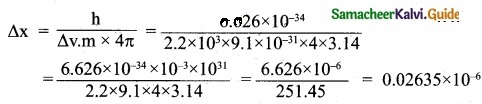∆x = 2.635 × 10-8
Uncertainty in position = 2.635 × 10-8

Question 27.
Distinguish between diffusion and effusion?
Diffusion:

1. Diffusion is the spreading of molecules of a substance throughout a space or a second subsance.
2. Diffusion refers to the ability of the gases to mix with each other.
3. E.g; Spreading of something such as brown tea liquid spreading through the water in a tea cup.

Effusion:

1. Effusion is the escape of gas molecules through a very small hole in a membrane into an evacuated area.
2. Effusion is a ability of a gas to travel through a small pin-hole.
3. E.g; Pouring out something like the soap studs bubbling out from a bucket of water.

Question 28.
For a chemical reaction the values of ∆H and ∆S at 300 K are – 10 kJ mol-1 and – 20 JK-1 mol-1 respectively. What is the value of ∆G of the reaction? Calculate the ∆G of a reaction at 600K assuming ∆H and ∆S values are constant. Predict the nature of the reaction?
Given:
∆H = -10 kJ mol-1 = -10000 J mol-1
∆S = – 20 JK-1 mol-1
T = 300 K

∆G?
∆G = ∆H – T∆S
∆G = -10 kJ mol-1 – 300 K × (-20 × 10-3) kJ K-1 mol-1
∆G = (-10 + 6) kJ mol-1
∆G = – 4 kJ mol-1

At 600 K,
∆G = – 10 kJ mol-1 – 600 K × (-20 × 10-3) k-1 mol-1
∆G = (-10 + 12) kJ mol-1
∆G + 2 kJ mol-1
The value of ∆G is negative at 300K and the reaction is spontaneous, but at 600K the value ∆G becomes positive and the reaction is non-spontaneous.Question 29.
For the reaction: A2(g) + B2(g) ⇄ 2AB(g); H is -∆ve.
The following molecular scenes represent different reaction mixture (A – light grey, B-dark grey)1. Calculate the equilibrium constant Kp and Kc.
2. For the reaction mixture represented by scene (x), (y) the reaction proceed in which directions?
3. What is the effect of increase in pressure for the mixture at equilibrium?Kc > Q i.e; forward reaction is favoured.

(III) Since ∆ng = 2 -2 = 0, thus, pressure has no effect. So by increasing the pressure, equilibrium will not be affected.Question 30.
Derive the relationship between the relative lowering of vapour pressure and mole fraction of the solute?Question 31.
Differentiate between the principle of estimation of nitrogen in an organic compound by

1. Dumas method
2. Kjeldahl’s method

1. Dumas method:
The organic compound is heated strongly with excess of CuO (Cupic Oxide) in an atmosphere of CO2 where free nitrogen, CO2 and H2O are obtained.

2. Kjeldahl’s method:
A known mass of the organic compound is heated strongly with cone. H2SO4, a little amount of potassium sulphate arid a little amount of mercury (as catalyst). As a result of reaction, the nitrogen present in the organic compound is converted to ammonium sulphate.Question 32.
In what way free radical affect the human body?

1. Free radicals can disrupt cell membranes.
2. Increase the risk of many forms of cancer.
3. Damage the interior lining of blood vessels.
4. Eads to a high risk of heart disease and stroke.

Question 33.
Dissolved oxygen in water is responsible for aquatic life. What processes are responsible for the reduction in dissolved oxygen in water?

1. Organic matter such as leaves, grass, trash can pollute water. Microorganisms present in water can decompose these organic matter and consume dissolved oxygen in water.
2. Eutrophication is a process by which water bodies receive excess nutrients that stimulates excessive plant growth.
3. This enhanced plant growth in water bodies is called algal bloom.
4. The growth of algae in extreme abundance covers the water surface and reduces the oxygen concentration in water. Thus, bloom-infeded water inhibits the growth of other living organisms in the water body.
5. This process in which the nutrient rich water support a dense plant population, kills animal life by depriving it of oxygen and results in loss of biodiversity is known as eutrophication.

PART – IV

Answer all the questions. [5 × 5 = 25]

Question 34 (a).
(I) Calculate the equivalent mass of sulphuric acid?
(II) The reaction between aluminium and ferric oxide can generate temperatures up to 3273 K and is used in welding metals.
(Atomic mass of Al = 27 u Atomic mass of O = 16 u)
2Al + Fe2O3 → Al2O3 + 2Fe; If, in this process, 324 g of aluminium is allowed to react with 1.12 kg of ferric oxide.
(a) Calculate the mass of Al2O3 formed.
(b) How much of the excess reagent is left at the end of the reaction?

[OR]

(b) (I) Consider the following electronic arrangements for the d5 configuration?

(a)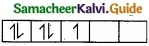(b)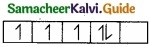(c)1. Which of these represents the ground state?
2. Which configuration has the maximum exchange energy?

(II) An ion with mass number 56 contains 3 units of positive charge and 30.4% more neutrons than electrons. Assign symbol to the ion?
(a) (I) Equivalent mass of sulphuric acid:
Sulphuric acid = H2SO4
Molar mass of Sulphuric acid = 2 + 32 + 64 = 96
Basicity of Sulphuric acid = 2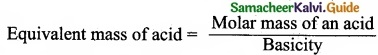= $$\frac{96}{2}$$ = 49 g eq-1

(II) (a)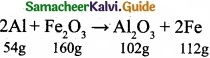As per balanced equation 54 g Al is required for 112 g of iron and 102 g of Al2O3.
54 g of Al gives 102 g of Al2O3.
∴ 324 g of Al will give $$\frac{102}{54}$$ × 324 = 612 g of AlcO3

(b) 54 g of Al requires 160 g of Fe2O3 for welding reaction.
∴ 324 g of Al will require $$\frac{160}{54}$$ × 324 = 960 g of Fe$$\frac{102}{54}$$O3
∴ Excess Fe2O3 -Unreacted Fe2O3 = 1120 – 960 = 160 g 160 g of exces reagent is left at the end of the reaction.

[0R]

(b)
(I) 1.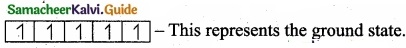2.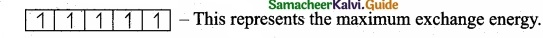(II) Let the no. of electrons in the ion = x
∴ The no. of the protons = x + 3 (as the ion has three units positive charge)
and the no. of neutrons = x + $$\frac{30.4x}{100}$$ = x + 0.304 x
Now, mass number of ion = Number of protons + Number of neutrons
= (x + 3) + (x + 0.304 x)
∴ 56 = (x + 3) + (x + 0.304 x) or 2.304 x = 56 – 3 = 53
x = $$\frac{53}{2.304}$$ = 23
Atomic number of the ion (or element) = 23 + 3 = 26
The element with atomic number 26 is iron (Fe) and the corresponding ion is Fe3+.Question 35 (a).
(I) State the Newland’s law of octaves?
(II) What are the two exceptions of block division in the periodic table?

[OR]

(b) (I) Complete the following reactions.
(a)(b) 2 BeCl2 + LiaH4 →?

(II) What happens when quick lime reacts with
(a) H2O and
(b) CO2?

(I) The Law of octaves states that, “when elements are arranged in the order of increasing atomic weights, the properties of the eighth element are a repetition of the properties of the first element”.

(II)

1. Helium has two electrons. Its electronic configuration is 1s2. As per the configuration, it is supposed to be placed in ‘s’ block, but actually placed in 18th group which belongs to ‘p’ block. Because it has a completely filled valence shell as the other elements present in 18th group. It also resembles with 18th group elements in other properties. Hence helium is placed with other noble gases.

2. The other exception is hydrogen. It has only one s-electron and hence can be placed in group 1. It can also gain an electron to achieve a noble gas arrangement and hence it can behave as halogens (17th group elements). Because of these assumptions, position of hydrogen becomes a special case. Finally, it is placed separately at the top of the periodic table.

[OR]

(b) (I) (a) Beryllium oxide is heated with carbon and chloride to get BeCl2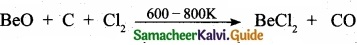(b) Beryllium chloride is treated with LiAlH4 to get beryllium hydride.
2BeCl2 + LiAlH4 → 2BeH2 + Licl + Alcl3

(II) (a) CaO + H2O → Ca(OH)2 (calcium hydroxide)
(b) CaO + CO2 → CaO3 (calcium carbonate)Question 36 (a).
(I) State the first law of thermodynamics?
(II) Calculate the enthalpy of combustion of ethylene at 300 Kc at constant pressure, if its heat of combustion at constant volume (∆U) is -1406 kJ?

[OR]

(b)
(I) Explain how the equilibrium constant Kc predict the extent of a reaction? (3)
(II) Explain about the effect of catalyst in an equilibrium reaction? (2)
(a) (I) The first law of thermodynamics states that “the total energy of an isolated system . remains constant though it may change from one form to another” (or) Energy can neither be created nor destroyed, but may be converted from one form to another.
(II) The complete ethylene combustion reaction can be written as,

C2H4(g) + 3O2(g) → 2CO2(g) + 2H2O(l)
ΔU = -1406 kJ
Δn = np(g) – nr(g)
Δn = 2 – 4 – 2
ΔH = ΔU + RTΔng
ΔH = -1406 + (8.314 × 10-3 × 300 × (-2)) ΔH = -1410.9 kJ

[OR]

(b) (I)

1. The value of equilibrium constant KC tells us the extent of the reaction i.e., it indicatehow far the reaction has proceeded towards product formation at a given temperature.
2. A large value of KC indicates that the reaction reaches equilibrium with high product yield on the other hand, lower value of KC indicates that the reaction reaches equilibrium with low product yield.
3. If KC > 103, the reaction proceeds nearly to completion.
4. If KC < 10-3 the reaction rarely proceeds.
5. It the KC is in the range 10-3 to 103, significant amount of both reactants and products are present at equilibrium.

(II) Addition of catalyst does not affect the state of equilibrium. The catalyst increases the rate of both the forward and reverse reactions to the same extent. Hence it does not change the equilibrium composition of the reaction mixture.Question 37 (a).
(I) Briefly explain geometrical isomerism in alkenes by considering 2- butene as an example.
2 – butene: Geometrical isomerism: CH3 – CH = CH – CH

(II) What is meant by condensed structure? Explain with an example.

[OR]

(b)
(I) Why cut apple turns a brown colour?
(II) Predict the product for the following reaction,

1.2.3.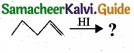(a) (I)

• Geometrical isomers are the stereoisomers which have different arrangement of groups or atoms around a rigid framework of double bonds. This type of isomerism occurs due to restricted rotation of double bonds or about single bonds in cyclic compounds.
• In 2-butene, the carbon-carbon double bond is sp2 hybridised. The carbon-carbon double bond consists of a s bond and a p bond. The presence of p bond lock the molecule in one position. Hence, rotation around C = C bond is not possible.
•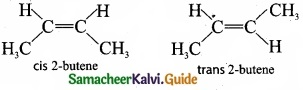• These two compounds are termed as geometrical isomers and are termed as cis and transform.
• The cis isomer is the one in which two similar groups are on the same side of the double bond. The trans isomer is that in which two similar groups are on the opposite side of the double bond. Hence, this type of isomerism is called cis-trans isomerism.

(II) The bond line structure can be further abbreviated by omittiilg all the these dashes representing covalent bonds and by indicating the number of identical groups attached to an atom by a subscript. The resulting expression of the compound is called a condensed structural formula.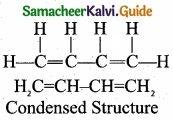[OR]

(b) (I)

1. Apples contains an enzyme called polyphenol oxidase (PPO) also known as tyrosinase.
2. Cutting an apple exposes its cells to the atmospheric oxygen and oxidizes the phenolic compounds present in apples. This is called the “enzymatic browning” that turns a cut apple brown.
3. In addition to apples, enzymatic browning is also evident in bananas, pears, avocados and even potatoes.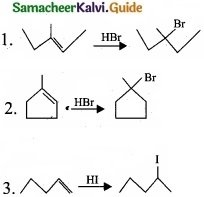Question 38 (a).
Suggest the route for the preparation of the following from benzene?

1. 3 – chloro-nitrobenzene
2. 4 – chlorotoluene
3. Bromobenzene
4. m – dinitrobenzene

[OR]

(b) A hydrocarbon C3H6 (A) reacts with HBr to form compound (B). Compound (B) reacts with aqueous potassium hydroxide to give (C) of molecular formula C3H6O. What are (A) (B) and (C). Explain the reactions?
(a)
1. Preparation of 3 – chloronitro – benzene from benzene: Benzene undergoes nitration and followed by chlorination and it leads to the formation of 3 – chloronitrobenzene.2. Preparation 4-chlorotoulene from benzene: Benzene undergoes Friedal craft’s alkylation followed by chlorination and it leads to the formation of 4 – chlorotoulene.3. Preparation of Bromobenzene from benzene: Benzene undergo bromination to give bromobenzene.4. Preparation of m-dinitrobenzene from benzene: Benzene undergo twice the time nitration to give m-dinitrobenzene.[OR]

(b)1. The hydrocarbon with molecular formula C3H6 (A) is identified as propene,
CH – CH = CH2
2. Propene reacts with HBr to form bromopropane CH3 – CH2 – CH2Br as (B).3. 1 – bromopropane react with aqueous potassium hydroxide to give 1 – propanol CH3 – CH2 – CH2OH as (C).
4. 2 – bromo propane reacts with aqueous KOH to give 2-propanol as (C)